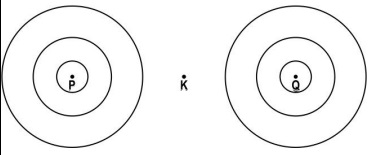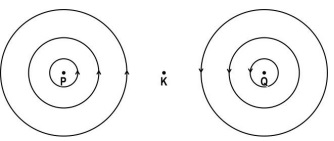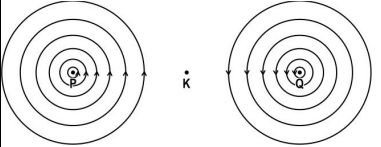Practice Questions CBSE - Science Class 10 (2023 Boards)

Class 10
Solutions to CBSE Sample Paper - Science Class 10

## P and Q represent two straight wires carrying equal current (I) in a direction perpendicular to the plane of the screen outwards. K is the midpoint of the line joining P and Q. The image shows the magnetic field lines around the wire. But the direction of the magnetic field is not marked.## (d) If B is the magnetic field at point K due to the current in wire P and the current in wire Q is reversed, what will be the magnetic field at midpoint K?

(a)(b) The strength of the magnetic field around P and Q will increase.(c) Zero.

The magnetic fields at point K due to current in the wires P and Q are equal in magnitude but opposite in direction. The two fields cancel each other.

(d)

If the current in Q is reversed , the direction of the magnetic field due to Q at K will reverse , to be in the same direction as P .

Thus, the two magnetic fields will be added up to 2B .

Get live Maths 1-on-1 Classs - Class 6 to 12